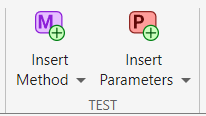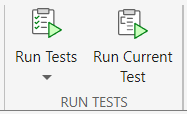## Insert Test Code Using Editor

MATLAB® lets you create and run test classes interactively. You can create a test class from a template, and then use the code insertion options of the toolstrip to add methods and parameterization properties to the class. You can choose whether to add a method or property at the test level, method-setup level, or class-setup level.

This example shows how to use the MATLAB Editor to write and run a simple parameterized test for a function. To set up the example, define the `cleanData` function in a file named `cleanData.m` in your current folder. The function accepts a numeric array and returns a cleaned and sorted version of the array. It vectorizes the array, removes the `NaN`, `0`, and `Inf` entries, and finally sorts the vector.

```function y = cleanData(X) y = X(:); % Vectorize the array y = rmmissing(y); % Remove NaN entries % Remove 0 and Inf entries idx = (y == 0 | y == Inf); y = y(~idx); % If the vector is empty, set it to eps if isempty(y) y = eps; end y = sort(y); % Sort the vector end```

### Create Test Class

To test the `cleanData` function, create a test class from a template. On the Editor tab, select New > Test Class. Name the class `CleanDataTest`, and save it in a file named `CleanDataTest.m` in your current folder.

The template provides a `TestClassSetup` `methods` block, a `TestMethodSetup` `methods` block, and a `Test` `methods` block that defines a simple `Test` method. The test class in this example requires one test-level parameterization property and two parameterized `Test` methods. Because it does not require any setup and teardown code, remove the `TestClassSetup` and `TestMethodSetup` `methods` blocks.

```classdef CleanDataTest < matlab.unittest.TestCase methods (Test) % Test methods function unimplementedTest(testCase) testCase.verifyFail("Unimplemented test"); end end end```

Starting in R2022b, when you save or open a test class definition file, the Test section appears on the Editor tab. Use this section to insert code that defines a method or parameterization property:

• To insert code that defines a `Test` method, click. To access the full list of options, click . These options let you add a method at the test level, method-setup level, or class-setup level. You can change the name of the method and implement it after it is inserted.

• To insert code that defines a test-level parameterization property, click. To access the full list of options, click . These options let you add a property at the test level, method-setup level, or class-setup level. You can change the name and value of the property after it is inserted.When you insert code for a method or property at the test level, method-setup level, or class-setup level, the code is added to the `methods` or `properties` block with the corresponding attribute. If the block does not exist, MATLAB creates it.

#### Add `data` Property

To test the `cleanData` function with different inputs, add a test-level parameterization property to the `CleanDataTest` class. With the test class code visible in the Editor, go to the Editor tab and in the Test section, click. MATLAB adds a property in a `properties` block with the `TestParameter` attribute. Rename the property as `data` and initialize it using a structure with four fields. The testing framework generates parameter names and values from the property value. For more information about parameterized tests, see Use Parameters in Class-Based Tests.

```classdef CleanDataTest < matlab.unittest.TestCase properties (TestParameter) data = struct("empty",[],"scalar",0, ... "vector",[13 NaN 0],"matrix",[NaN 2 0; 1 Inf 3]); end methods (Test) % Test methods function unimplementedTest(testCase) testCase.verifyFail("Unimplemented test"); end end end```

#### Add `sortTest` Method

The test class template includes a simple `Test` method named `unimplementedTest`. Modify this method to test an aspect of the `cleanData` function:

1. Rename the method as `sortTest`.

2. Parameterize the method by passing the `data` property as the second input argument to the method.

3. Add code to the method to verify that the `cleanData` function correctly sorts the array passed to it.

```classdef CleanDataTest < matlab.unittest.TestCase properties (TestParameter) data = struct("empty",[],"scalar",0, ... "vector",[13 NaN 0],"matrix",[NaN 2 0; 1 Inf 3]); end methods (Test) % Test methods function sortTest(testCase,data) actual = cleanData(data); testCase.verifyTrue(issorted(actual)) end end end```

#### Add `nonemptyTest` Method

To test if the `cleanData` function returns a nonempty value, add another `Test` method to the class by clickingin the Test section. Implement the method by following these steps:

1. Rename the method as `nonemptyTest`.

2. Parameterize the method by passing the `data` property as the second input argument to the method.

3. Add code to the method to verify that the `cleanData` function returns a nonempty value.

Save the file. This code provides the complete contents of the `CleanDataTest` class.

```classdef CleanDataTest < matlab.unittest.TestCase properties (TestParameter) data = struct("empty",[],"scalar",0, ... "vector",[13 NaN 0],"matrix",[NaN 2 0; 1 Inf 3]); end methods (Test) % Test methods function sortTest(testCase,data) actual = cleanData(data); testCase.verifyTrue(issorted(actual)) end function nonemptyTest(testCase,data) actual = cleanData(data); testCase.verifyNotEmpty(actual) end end end```

### Run Tests in Test Class

You can run the tests in the `CleanDataTest` class interactively in the Editor or in the Test Browser app. For example, with the test class code visible in the Editor, go to the Editor tab and in the Run section, click. In this example, all the tests pass.For more information on how to run tests and customize your test run interactively, see Run Tests in Editor and Run Tests Using Test Browser.